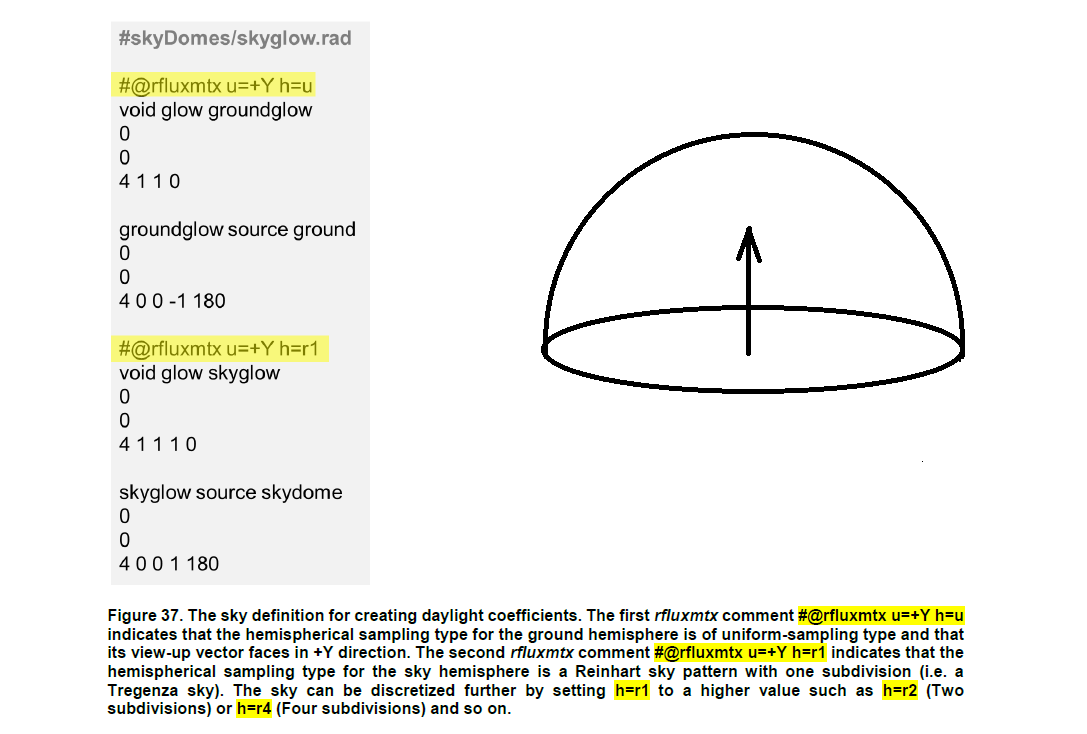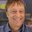Question-and-Answer Resource for the Building Energy Modeling Community
Get s tarted with the Help page

# What is the "up" direction defined by -u for the sample hemisphere of the rfluxmtx program

In the tutorial Daylighting Simulations with Radiance using Matrix-based Methods (pp. 42), the "view-up" vector of the rfluxmtx hemispherical sampling is set to +Y direction, as in the figure below.

To my understanding, the window of the room model is facing the negative Y direction, which is the "south" direction in Radiance. Then, why is the sampling hemisphere taken to the positive Y direction here? In other words, shouldn't the sampling rays be shot into the negative Y directions to get the daylight matrix?

How to understand the definition of the "view-up" vector?.

edit retag close merge delete

Sort by » oldest newest most votedThe "up" direction for the hemisphere orients the coordinate system. In this case, setting "up" to +Y is the same as "north" by gensky conventions. You can of course define it in another way, but other parts of your calculation will then have to change, such as the way you use genskyvec. The number of samples will be distributed the same whatever you choose for "+Y" as their density is radially symmetric (or nearly so) for all hemisphere types.

I hope this helps.

more

Thank you. Howeve, I am still confused about the -u option.

For example, if I have three buildings, one with the window facing the south (-Y direction), the second with the window facing the east (+X direction), and the third with the window facing upward (+Z direction). When I solve for the daylight matrix from the sky to the three windows, should I use u = Y, u = X and u = Z respectively in the rfluxmtx comments of the skyglow definition or does it matter?

I think you may be confusing the "up" direction with the hemisphere normal, which is determined from the surface normal, not the "up" vector. The arrow in Figure 37 is the surface normal. The up vector determines azimuthal rotation of the hemisphere, not the normal direction. In the case of your 3 windows, the surface normal of the window panes will define the hemisphere orientation, and the up direction can be "+Z" for the south- and east-facing windows, and should probably be set to "+Y" for the skylight. Does this make sense?

I see. The 'up' direction is the zero-azimuth (which is North) direction of the hemisphere, right? In this case, it makes sense to align the 'up' vector with the Y axis of the model when sampling the sky dome, as the gensky convention puts North in the Y axis.